# Stereo 175 watt amplifier or 350 watt mono amplifier STA517B350 watt mono amplifier
Stereo circuit in datasheet here
Read More...

# Stereo 300W Audio Amplifier Circuit Diagram LM SeriesLM 4702BTA

Feature of LM 4702BTA
Very High Voltage Operation
Scalable Output Power
Minimum External Components
External Compensation
Thermal Shutdown and Mute

Typical Audio Amplifier Application Circuit LM 4702BTAStereo 300W Audio Amplifier Circuit Diagram LM Series LM4702BTA

Read More...

# Up to 1000W Power amplifier TP2350B

Features:
• Class-T architecture
• Pin compatible with Tripath TK2150
• Proprietary Digital Power Processing technology
• "Audiophile" Sound Quality
• 0.02% THD+N @ 50W, 8Ω
• 0.03% IHF-IM @ 30W, 8Ω
• High Efficiency
• 95% @ 150W @ 8Ω
• 90% @ 275W @ 4Ω
• Supports wide range of output power levels
• Up to 300W/channel (4Ω), single-endedoutputs
• Up to 1000W (4Ω), bridged outputs
• Output over-current protection
• Over- and under-voltage protection
• Over-temperature protection
Application
• Audio/Video Amplifiers & Receivers
• Pro-audio Amplifiers
• Automobile Power Amplifiers
• Subwoofer Amplifiers
Circuit Diagram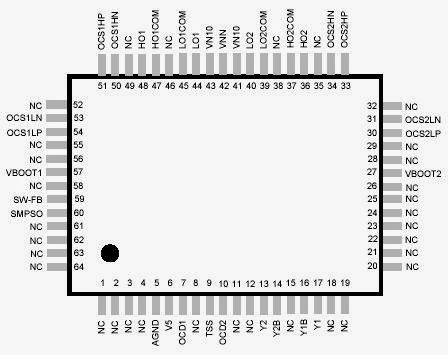Up to 1000W Power amplifier TP2350B

Read More...

# 2 x 210 Watt STEREO DIGITAL AMPLIFIER POWER STAGE

• 2×160 W at 10% THD+N Into 8-Ω BTL
• 2×210 W at 10% THD+N Into 6-Ω BTL
• 1×300 W at 10% THD+N Into 4-Ω PBTL2 x 210 Watt STEREO DIGITAL AMPLIFIER POWER STAGE

Read More...

# TP2150B 2 x 200 watt power amplifier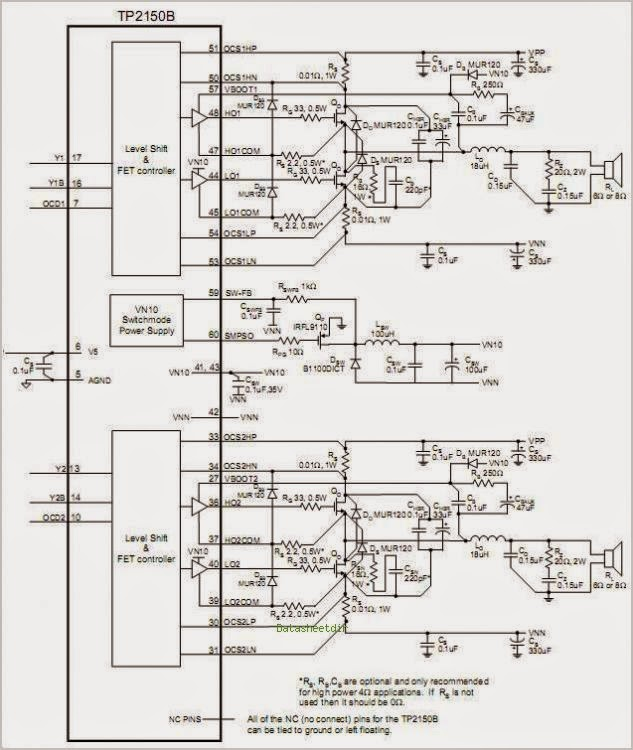Circuit diagram for TP2150B 2 x 200 watt power amplifier

Read More...

# 180W + 180W Power Supply Audio Power Amplifier ICs

Overview:

The STK412-000 series are class H audio power amplifier hybrid ICs that feature a built-in shift power supply circuit. These ICs provide high efficiency audio power amplification by controlling (switching) the supply voltage supplied to the power transistors according to the detected level of the input audio signal.

Features:

• Pin compatible IC series that covers power ratings from 50 W × 2 channels to 180 W × 2 channels at 0.7 or 0.8% THD, 20 Hz to 20 kHz. This allows the use of a common PCB for all output classes.
• The pin arrangement is also unified with that of the three-channel STK413-000 series. This means that PCBs designed for three-channel models can also be used for two-channel models.
• Miniature package — 50 W/ch to 120 W/ch (THD = 0.8%, f = 20 Hz to 20 kHz): 64 × 36.5× 8.5 mm* — 150 W/ch to 180 W/ch (THD = 0.7%, f = 20 Hz to 20 kHz): 78 × 44× 9 mm* * Not including the IC pins.
• Allowable load shorted time: 0.3 s
Circuit Diagram:

Read More...

# Two-Channel Shift Power Supply Audio Power Amplifier ICs 180W + 180 W

Overview The STK412-000 series are class H audio power amplifier hybrid ICs that feature a built-in shift power supply circuit. These ICs provide high efficiency audio power amplification by controlling (switching) the supply voltage supplied to the power transistors according to the detected level of the input audio signal.Power Sourse of  Two-Channel Shift Power Supply Audio Power Amplifier ICs 180W + 180 W

Circuit diagram and other info view
Read More...

# Baxandall Tone-Control Audio Amplifier

This circuit exploits the high slew rate, high input impedance, and high output-voltage capability of CA3140 BiMOS op amp. It also provides mid-band unity gain with standard linear potentiometers.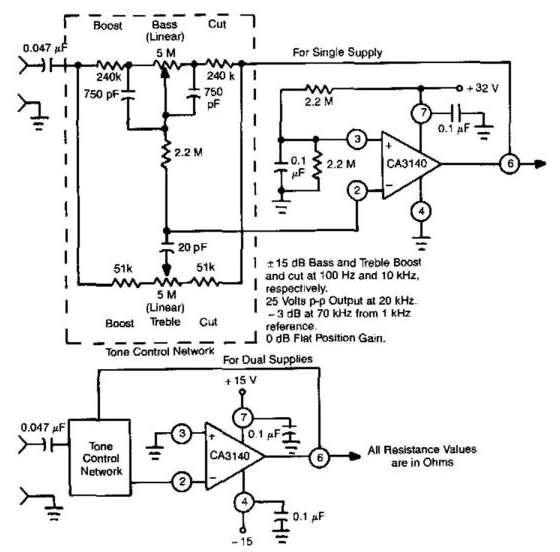Baxandall Tone-Control Audio Amplifier

Read More...

# Race-car-motor-crash

The mixer can be multiplexed between the SLF and VCO functions.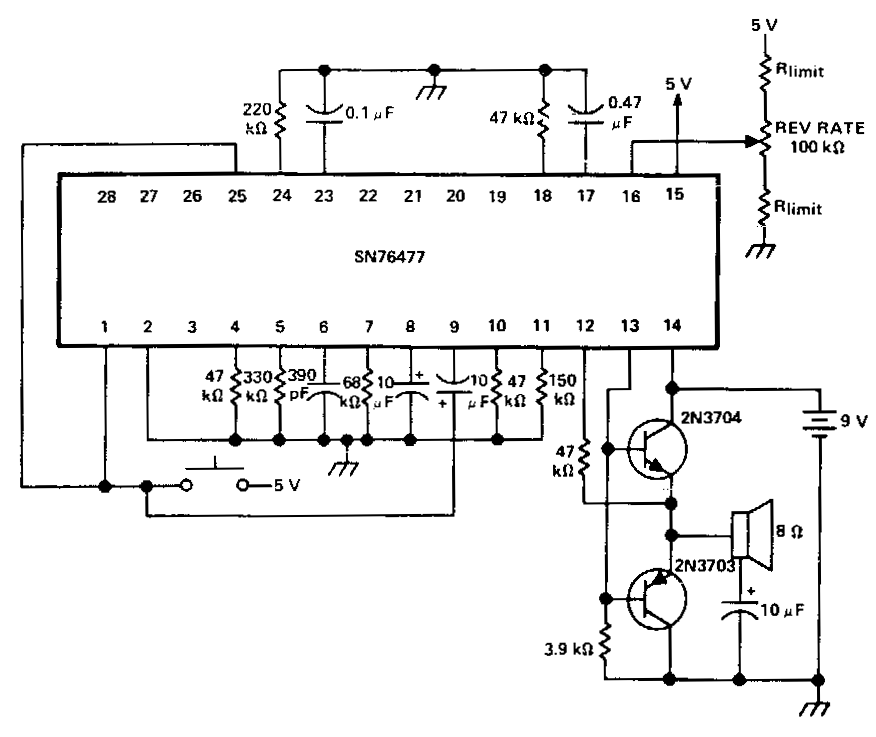For two simultaneous race-car sounds.
Read More...

# How to Build a Subwoofer Box

Building a subwoofer box is the foundation of your new car audio system . In this video I will show you different techniques that will make your sub enclosure perfect and help it sound even better!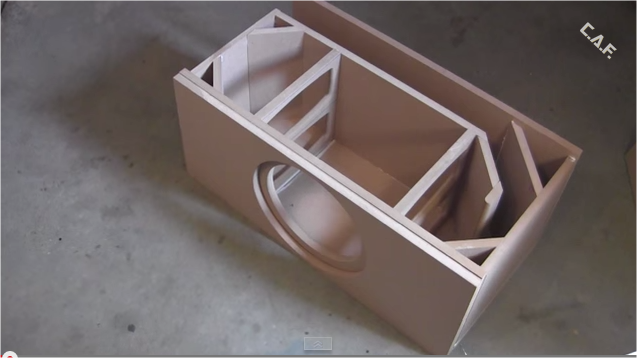How to Build a Subwoofer Box

Read More...

# 300W Building a subwoofer enclosure

This is how a subwoofer enclosure is built, it is ported and is tuned to 35hz the subwoofers that i am installing are 2 re 8's that are dual voice coil and i am pushing 300watts at 4ohms.300W Building a subwoofer enclosure
Read More...

# 7 segment display Arduino

The aim of this article demonstrate how the Arduino can run on 7-segment display. We used a SA56-11GWA which is a single display with common anode, it means that the anode is common to all digits, and to the point.
A display consists of 8 LEDs, 7 form the figure and 1 point decimal.A conversion between decimal and what we intend to show the binary information that you receive is called the BCD (Binary Coded Decimal).

In terms of hardware, each LED work individually, so each of the segments has to lead a limiting resistor, in this case, each digit has an operating voltage of 2.2V and consumes 20mA. If the voltage is 5volts, we need the resistor fall 5-2.2 = 3.8V, by Ohm's Law we can calculate, R = V res / I led R = 3.8 / 0.020 = 190 Ω +/- 20% (let's use a resistor with 220Ω). This applies to this display SA56-11GWA used as an example, other values ​​may be different, it is useful to calculate the required resistance value.
DecimalThe321
00000
10001
20010
30011
40100
50101
60110
70111
81000
91001
The first thing we have to do is convert our decimal number to binary, we will use examples only 0-9 this first phase
``` decToBcd byte (byte val)
{
return ((val / 10 * 8) + (val% 10));
}
```
This makes our decimal value to binary, for example, assuming that have numbers with more than two digits, 27 will be 0010 0111 (2 - 7 BCD). If we have only one figure 2 for example, will have 0010.
This allows us to act on an IC decoder (4511) and the use of only four BCD outputs Arduino, so can convert a result of a count value, or for any input digit.
``` / *
Example of a counter from 0 to 9 that adds each
Since there is an entry in A0

author: Arturo Guadalupi
* /

// Declaration of pins used in the 4511 entries
The const int = 2;
const int b = 3;
const int c = 4;
const int D = 5;

void setup () {
pinMode (A0, INPUT);
pinMode (A, OUTPUT);  // LSB
pinMode (B, OUTPUT);
pinMode (C, OUTPUT);
pinMode (D, OUTPUT);  // MSB

}

int count = 0;  // Used for the variable number

void loop () {
if (digitalRead (A0) == LOW) // if the button is pressed on the A0 input
{
count ++;
delay (200);  // The delay prevents multiple counts
if (count == 10) // 0-9 counter
count = 0;
to_BCD ();  // Convert to binary
}

if (count == 10)
count = 0;

}

to_BCD void ()
{
if (count == 0) // write 0000
{
digitalWrite (A, LOW);
digitalWrite (B, LOW);
digitalWrite (C, LOW);
digitalWrite (D, LOW);
}

if (count == 1) // write 0001
{
digitalWrite (A, HIGH);
digitalWrite (B, LOW);
digitalWrite (C, LOW);
digitalWrite (D, LOW);
}

if (count == 2) // write 0010
{
digitalWrite (A, LOW);
digitalWrite (B, HIGH);
digitalWrite (C, LOW);
digitalWrite (D, LOW);
}

if (count == 3) // write 0011
{
digitalWrite (A, HIGH);
digitalWrite (B, HIGH);
digitalWrite (C, LOW);
digitalWrite (D, LOW);
}

if (count == 4) // write 0100
{
digitalWrite (A, LOW);
digitalWrite (B, LOW);
digitalWrite (C, HIGH);
digitalWrite (D, LOW);
}

if (count == 5) // write 0101
{
digitalWrite (A, HIGH);
digitalWrite (B, LOW);
digitalWrite (C, HIGH);
digitalWrite (D, LOW);
}

if (count == 6) // write 0110
{
digitalWrite (A, LOW);
digitalWrite (B, HIGH);
digitalWrite (C, HIGH);
digitalWrite (D, LOW);
}

if (count == 7) // write 0111
{
digitalWrite (A, HIGH);
digitalWrite (B, HIGH);
digitalWrite (C, HIGH);
digitalWrite (D, LOW);
}

if (count == 8) // write 1000
{
digitalWrite (A, LOW);
digitalWrite (B, LOW);
digitalWrite (C, LOW);
digitalWrite (D, HIGH);
}

if (count == 9) // write 1001
{
digitalWrite (A, HIGH);
digitalWrite (B, LOW);
digitalWrite (C, LOW);
digitalWrite (D, HIGH);
}
} ```

## Convert decimal to binary (8bit)

It is possible to directly transfer the binary value for the exits, here is an example of cyclic counter zero to nine
``` ledpins int [] = {4,5,6,7,8,9,10,11};
byte digits [] = {B11111100, B01100000, B11011010, B11110010, B01100110, B10110110,
B10111110, B11100000, B11111110, B11110110};

void setup ()
{
for (int i = 0; i <8; i ++)
{
pinMode (ledpins [i] OUTPUT);
digitalWrite (ledpins [i], HIGH);
}
}

void loop ()
{
for (int i = 0; i <10; i ++)
{
displayDigit (i);
delay (1000);
AllOff ();
delay (500);
}
}

displayDigit void (int digit)
{
for (int i = 0; i <8; i ++)
{
digitalWrite (ledpins [i] ,! bitRead (digits [Scan], 7-i));
}
}

void AllOff ()
{
for (int i = 0; i <8; i ++)
{
digitalWrite (ledpins [i], HIGH);
}
}
```
In this case not used the BCD outputs but a direct binary code in 8 bits (one for each connected LED)
We used a level 1 (HIGH) when the segment lights and 0 (LOW) when deleted. The last digit is always a 0 represents the decimal point that is not being used.

The AllOff () procedure off all digits reversing the common pin.

displayDigit () connects the right thread for each digit. The function bitread reads the bits of the binary number, starting with the rightmost bit.That's why the 7-i is used. When using the digitalWrite () statement, we can write 1 when we want to HIGH and LOW 0 when we want. This is the opposite of how our binary numbers are working. The operator! inverts the value. via electronica-pt.com
Read More...